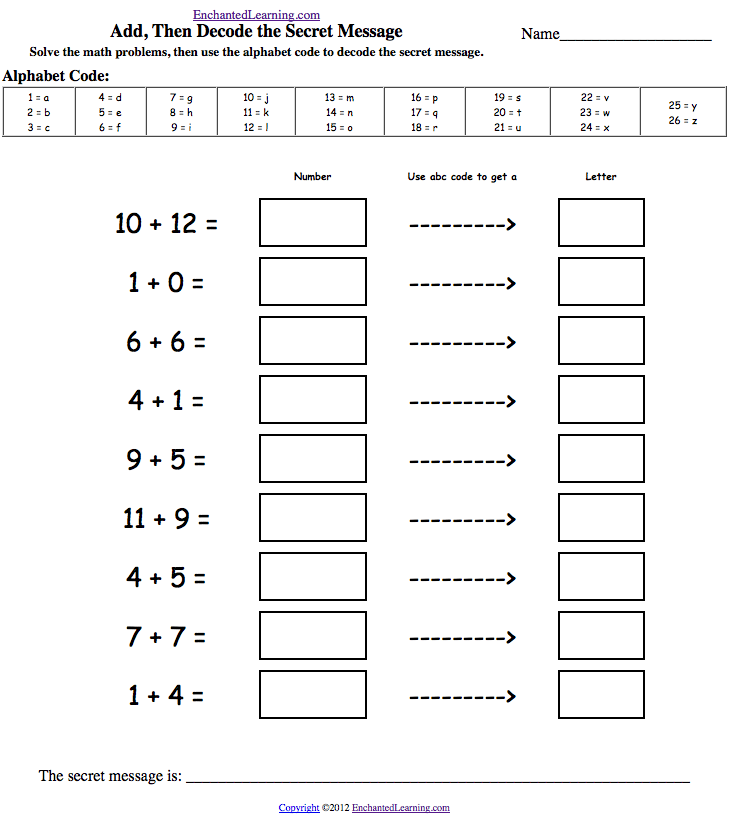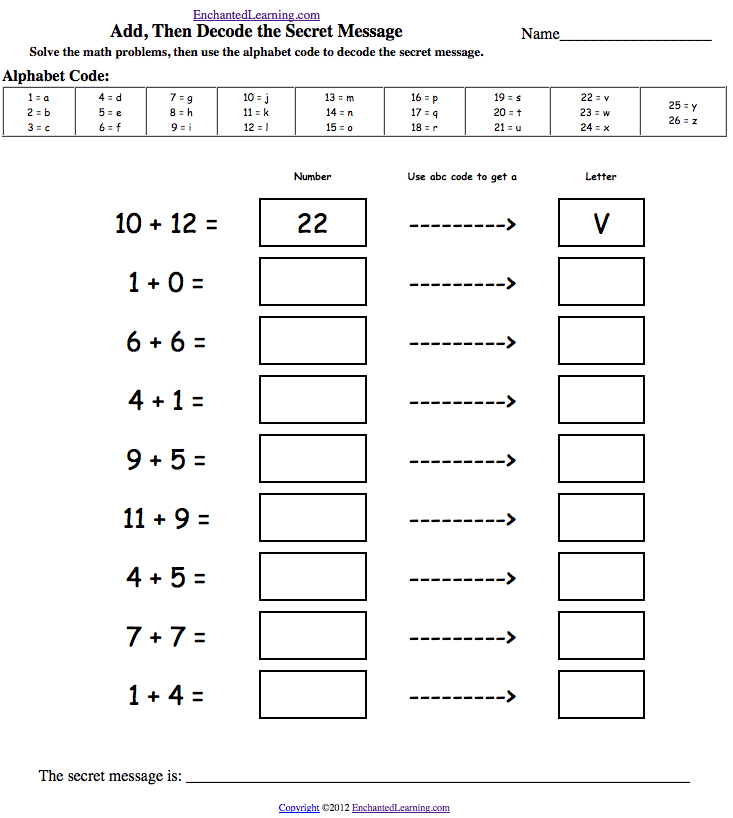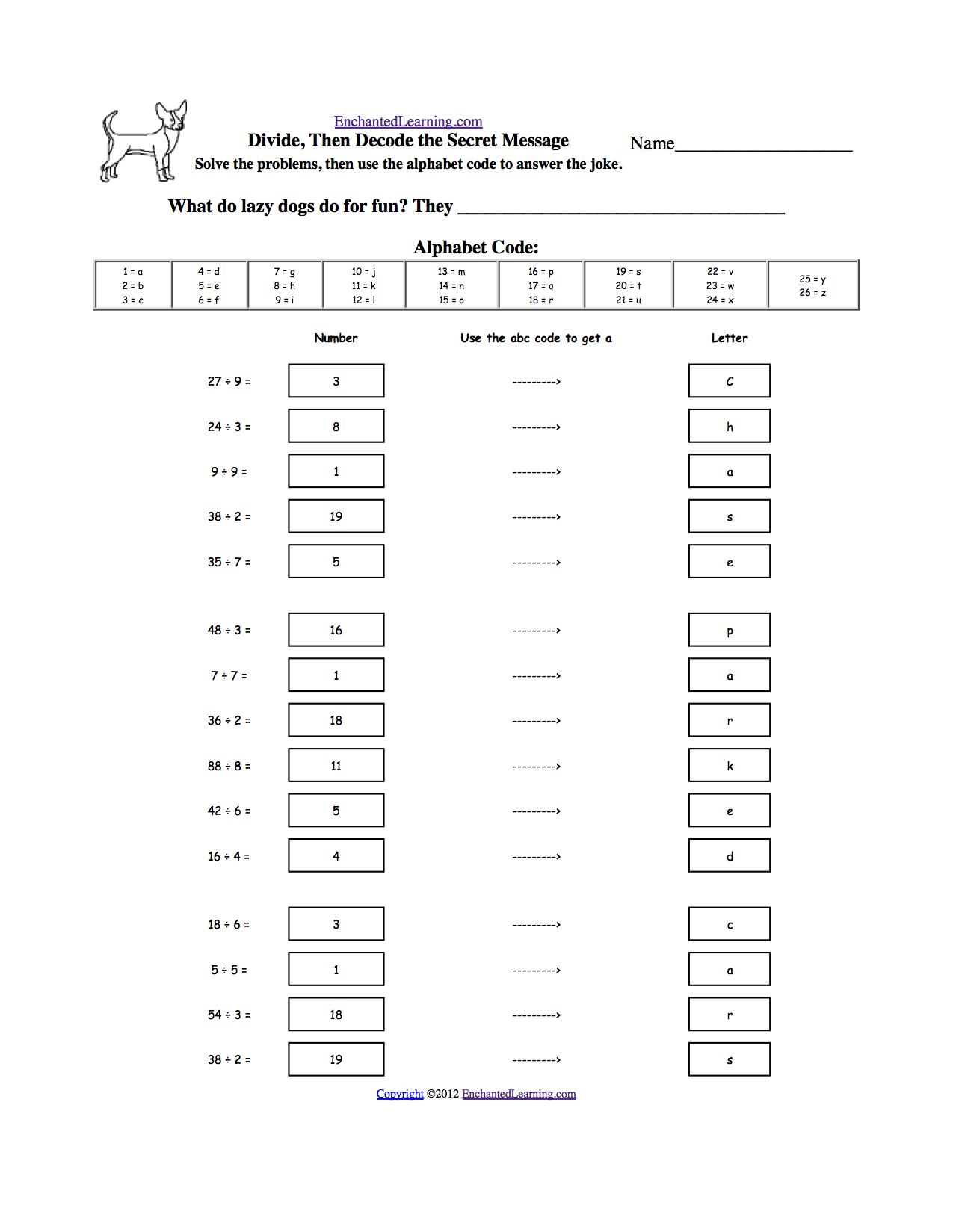EnchantedLearning.com is a user-supported site.
As a bonus, site members have access to a banner-ad-free version of the site, with print-friendly pages.

 You might also like: Clown Joke: Add and Subtract, Then Decode the Riddle: Arithmetic Worksheet Envelope Joke: Multiply, Then Decode: Arithmetic Worksheet Solve, Then Decode: Addition and Subtraction Worksheets Calendar Joke: Add and Subtract, Then Decode the Riddle: Arithmetic Worksheet Breakfast Joke: Subtract, Then Decode the Riddle: Arithmetic Worksheet Today's featured page: Animal Jokes and Riddles for Kids
 More Math EnchantedLearning.comSolve, Then Decode:Arithmetic Worksheets In these theme-based printable puzzles, the student first solves simple arithmetic problems, then uses the alphabet code to decode a riddle, quote, or secret message. Alphabet PagesAnimal Keeping Time:Add, then Decode the RiddleSolve the addition problems, then use the alphabet code to decode the answer to the animal riddle, "Which animal keeps the best time?" (Answer = A watchdog). Or go to the answer page.Abraham Lincoln:Add, then Decode the QuoteSolve the addition problems, then use the alphabet code to decode the end of the Abraham Lincoln quote, "I do not think much of a man who is not wiser today than he _______." (Answer = was yesterday). Or go to the answer page.Ocean:Add, then Decode the RiddleSolve the addition problems, then use the alphabet code to decode the answer to the riddle, "What did the ocean say to the shore?" (Answer = Nothing, it waved). Or go to the answer page.One Hundred Days of School:Add, then Decode the Secret MessageSolve the addition problems, then use the alphabet code to decode the secret message, "One hundred." Or go to the answer page.Plant a Light Bulb:Add, then Decode the RiddleSolve the addition problems, then use the alphabet code to decode the answer to the riddle, "Why did the gardener plant a light bulb? To grow _____." (Answer = a power plant). Or go to the answer page.St. Patrick's Day Riddle:Add, then Decode the RiddleSolve the addition problems, then use the alphabet code to solve the St. Patrick's Day riddle, "What happens when a leprechaun falls into a river?" Answer: "He gets wet." Or go to the answer page.Toothy Riddle:Add, then Decode the RiddleSolve the addition problems, then use the alphabet code to solve the toothy riddle, "What has many teeth but cannot eat?" Answer: "A comb." Or go to the answer page.Valentine's Day:Add, then Decode the Secret MessageSolve the addition problems, then use the alphabet code to decode the secret message, "Valentine." Or go to the answer page.Washington:Add, then Decode the RiddleSolve the addition problems, then use the alphabet code to decode the answer to the riddle, "What do you call doing 2,000 pounds of laundry?" (Answer = Washington). Or go to the answer page.

Subtraction WorksheetsApril Fool's Day:Subtract, then Decode the Secret MessageSolve the subtraction problems, then use the alphabet code to decode the April Fool's Day secret message, slooflirpa (April Fools spelled backwards). Or go to the answer page.Breakfast Joke:Subtract, then Decode the RiddleSolve the subtraction problems, then use the alphabet code to answer the breakfast riddle, "What can't you eat for breakfast?" Answer - Lunch and dinner. Or go to the answer page.Dalmatian Joke:Subtract, then Decode the RiddleSolve the subtraction problems, then use the alphabet code to answer the dog riddle, "Why can't Dalmatians win hide and seek?" Answer - They're always spotted. Or go to the answer page.Fish Joke:Subtract, then Decode the RiddleSolve the subtraction problems, then use the alphabet code to answer the fish riddle, "What part of a fish weighs the most?" Answer - The scales. Or go to the answer page.Pirate Joke:Subtract, then Decode the RiddleSolve the subtraction problems, then use the alphabet code to answer the pirate riddle, "How much did the pirate pay for his earrings?" Answer - A buccaneer. Or go to the answer page.Snowman and Wolf:Subtract, then Decode the RiddleSolve the subtraction problems, then use the alphabet code to answer the snowman and the wolf riddle, "What do you get when you cross a snowman and a wolf?" Answer - frostbite. Or go to the answer page.St. Patrick's Day:Subtract, then Decode the RiddleSolve the subtraction problems, then use the alphabet code to answer the St. Patrick's Day riddle, "What do you call a fake stone in Ireland?" Answer - A sham rock. Or go to the answer page.Valentine's Day:Subtract, then Decode the RiddleSolve the subtraction problems, then use the alphabet code to answer the Valentine's Day riddle, "What do you call a very small valentine?" Answer - A valentiny. Or go to the answer page.Banana Shoe Riddle: Add and Subtract, then Decode the RiddleSolve the addition and subtraction problems, then use the alphabet code to answer the silly riddle, "What do you call a shoe made from banana peels? (Answer - A slipper). Or go to the answer page.Clown Riddle: Add and Subtract, then Decode the RiddleSolve the addition and subtraction problems, then use the alphabet code to answer the clown riddle, "Why did the clown go to the doctor?" (Answer - He was feeling funny). Or go to the answer page.Leopard Riddle: Add and Subtract, then Decode the RiddleSolve the addition and subtraction problems, then use the alphabet code to answer the leopard riddle, "Which side of a leopard has the most spots?" (Answer - The outside). Or go to the answer page.Man-in-the-Moon Riddle: Add and Subtract, then Decode the RiddleSolve the addition and subtraction problems, then use the alphabet code to answer the man-in-the-moon riddle, "How does the man-in-the-moon cut his hair?" (Answer - Eclipse it). Or go to the answer page.Owl Riddles:Add and Subtract, then Decode the RiddlesSolve the addition and subtraction problems, then use the alphabet code to answer the owl riddles, "What do you call an owl with a deep voice?" (Answer - A growl), and "Someone said you sound like an owl." (Answer - Who?). Or go to the answer page.Penguin Money Riddle:Add and Subtract, then Decode the RiddleSolve the addition and subtraction problems, then use the alphabet code to answer the penguin riddles, "Where do penguins keep their money?" (Answer - In a snow bank). Or go to the answer page.Raining Cats and Dogs Riddle: Add and Subtract, then Decode the RiddleSolve the addition and subtraction problems, then use the alphabet code to answer the weather riddle, "What's worse than raining cats and dogs? (Answer - Hailing taxis). Or go to the answer page.Snow Boots Riddle: Add and Subtract, then Decode the RiddleSolve the addition and subtraction problems, then use the alphabet code to answer the snow boots riddle, "What's the biggest problem with snow boots? (Answer - They melt). Or go to the answer page.Twenty-eight Days Riddle: Add and Subtract, then Decode the RiddleSolve the addition and subtraction problems, then use the alphabet code to answer the calendar riddle, "How many months have 28 days? (Answer - All of them). Or go to the answer page.US Constitution Riddle: Add and Subtract, then Decode the RiddleSolve the addition and subtraction problems, then use the alphabet code to answer the US Constitution riddle, "Where was the US Constitution signed? (Answer - At the bottom). Or go to the answer page.

Multiplication WorksheetsEarth Joke:Multiply, then Decode the RiddleSolve the multiplication problems, then use the alphabet code to decode the answer to the Earth riddle, "What's in the middle of Earth?" (Answer = The letter "r"). Or go to the answer page.Egg Joke:Multiply, then Decode the RiddleSolve the multiplication problems, then use the alphabet code to decode the answer to the egg riddle, "Why did the egg hide?" (Answer = He was a little chicken.) Or go to the answer page.Envelope Joke:Multiply, then Decode the RiddleSolve the multiplication problems, then use the alphabet code to decode the answer to the envelope riddle, "What word starts with an "e", ends with an "e", but has only one letter?" (Answer = An envelope). Or go to the answer page.Ocean Rock Joke:Multiply, then Decode the RiddleSolve the multiplication problems, then use the alphabet code to decode the answer to the rock riddle, "What kind of rocks are never found in the ocean?" (Answer = Dry ones). Or go to the answer page.

Division WorksheetsCalendar Joke:Divide, then Decode the RiddleSolve the division problems, then use the alphabet code to decode the answer to the calendar riddle, "When does Friday come before Thursday?" (Answer = In the dictionary). Or go to the answer page.Dentist Appointment Joke:Divide, then Decode the RiddleSolve the division problems, then use the alphabet code to decode the answer to the dentist riddle, "What is the best time for a dentist appointment?" (Answer = Two thirty). Or go to the answer page.Mixed-Up Hen Joke:Divide, then Decode the RiddleSolve the division problems, then use the alphabet code to decode the answer to the chicken riddle, "What did the mixed-up hen lay?" (Answer = Scrambled eggs). Or go to the answer page.Lazy Dog Joke:Divide, then Decode the RiddleSolve the division problems, then use the alphabet code to decode the answer to the animal riddle, "What do lazy dogs do for fun?" (Answer = They chase parked cars). Or go to the answer page.Shamrock Joke:Divide, then Decode the RiddleSolve the division problems, then use the alphabet code to decode the answer to the St. Patrick's Day riddle, "Why do people wear shamrocks on St. Patrick's Day?" (Answer = Real rocks are too heavy). Or go to the answer page.

Enchanted Learning®
Over 35,000 Web Pages
Sample Pages for Prospective Subscribers, or click below

 Overview of Site What's New Enchanted Learning Home Monthly Activity Calendar Books to Print Site Index K-3 Crafts K-3 Themes Little ExplorersPicture dictionary PreK/K Activities Rebus Rhymes Stories Writing Cloze Activities Essay Topics Newspaper Writing Activities Parts of Speech Fiction The Test of Time iPhone app TapQuiz Maps - free iPhone Geography Game Biology Animal Printouts Biology Label Printouts Biomes Birds Butterflies Dinosaurs Food Chain Human Anatomy Mammals Plants Rainforests Sharks Whales Physical Sciences: K-12 Astronomy The Earth Geology Hurricanes Landforms Oceans Tsunami Volcano Languages Dutch French German Italian Japanese (Romaji) Portuguese Spanish Swedish Geography/History Explorers Flags Geography Inventors US History Other Topics Art and Artists Calendars College Finder Crafts Graphic Organizers Label Me! Printouts Math Music Word Wheels

## Enchanted Learning Search

 Search the Enchanted Learning website for: